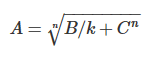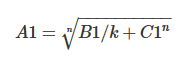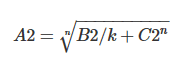# Finding the constants in an expression

• I
• ArnoldEdv

#### ArnoldEdv

I have a problem with this formula:I have two known value series: A1, B1, C1 and A2, B2, C2.
That gives me two equations with two unknowns n and k.Mentor note:
More readable versions of the two equations:
$$A_1 = \sqrt[n]{\frac{B_1} k + C_1^n}$$
and
$$A_2 = \sqrt[n]{\frac{B_2} k + C_2^n}$$
Does anyone have a clue how to solve this?

#### Attachments

Last edited by a moderator:
ArnoldEdv said:
I have a problem with this formula:
View attachment 230078
I have two known value series: A1, B1, C1 and A2, B2, C2.
That gives me two equations with two unknowns n and k.
View attachment 230079 View attachment 230080
Does anyone have a clue how to solve this?
You get a function ##k=k(n)## from the equations and then ##A_1^n-C_1^n=\gamma (A_2^n-C_2^n)## which I assume can only be solved numerically.

•jedishrfu
note: ##\gamma=\frac{B_1}{B_2}##.